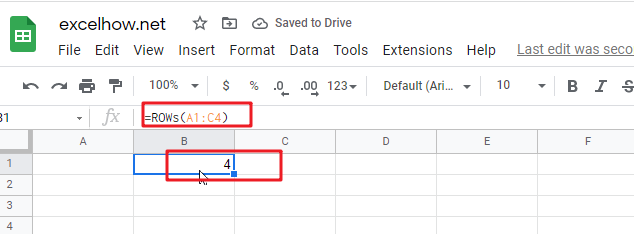# ExcelHow

This post will guide you how to use Google Sheets ROWS function with syntax and examples.

## Description

The Google Sheets ROWS function counts the number of rows in a cell reference.

The ROWS function can be used to return the number of rows in a specified range in google sheets. The purpose of this function is to get the number of rows in an array or cell reference and It’s returned value is a number of rows.

The ROWS function is a build-in function in Google Sheets and it is categorized as a LOOKUP function.

## Syntax

The syntax of the ROWS function is as below:

= ROWS(array)

Where the ROWS function arguments is:

• array-This is an required argument. An array, or A reference to a range of cells.

Note:

• ROWS just takes one argument, it can be a range or a array.
• Array can be a range or a cell reference
• If you want to count columns, you can use the COLUMNS function.
• If you want to get row numbers, you can use the ROW function.

## Google Sheets ROWS Function Examples

The below examples will show you how to use google sheets ROWS Function to return the number of rows in a range of cells.

#1 To get the number of rows in the cell range “A1:C4”, just using the following ROWS formula:

=ROWS(A1:C4)